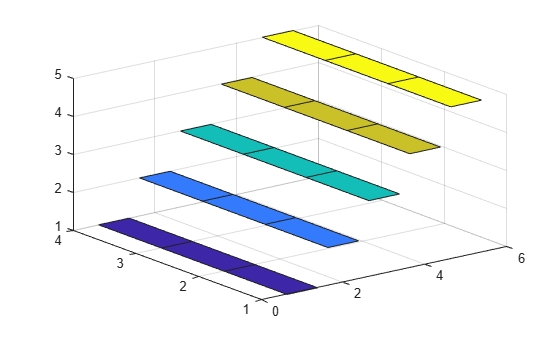ribbon

•Syntax

ribbon(Y)
ribbon(X,Y)
ribbon(X,Y,width)
ribbon(axes_handle,...)
h = ribbon(...)

Description

ribbon(Y) plots the columns of Y as three-dimensional ribbons of uniform width using X = 1:size(Y,1). Ribbons advance along the x-axis centered on tick marks at unit intervals, three-quarters of a unit in width. Ribbon maps values in X to colors in colormap linearly. To change ribbon colors in the graph, change the colormap.

ribbon(X,Y) plots three dimensional ribbons for data in Y, centered at locations specified in X. X and Y are vectors or matrices of the same size. Additionally, X can be a row or a column vector, and Y a matrix with length(X) rows. When Y is a matrix, ribbon plots each column in Y as a ribbon at the corresponding X location.

ribbon(X,Y,width) specifies the width of the ribbons. The default is 0.75. If width = 1, the ribbons touch, leaving no space between them when viewed down the z-axis. If width > 1, ribbons overlap and can intersect.

ribbon(axes_handle,...) plots into the axes with handle axes_handle instead of the current axes (gca).

h = ribbon(...) returns a vector of handles to surface graphics objects. ribbon returns one handle per strip.

Examples

collapse all

Create a ribbon plot of the peaks function.

[x,y] = meshgrid(-3:.5:3,-3:.1:3);
z = peaks(x,y);

figure
ribbon(y,z)